# Planimetrics - math word problems

Study plane measurements, including angles, distances, and areas. In other words - measurement and calculation of shapes in the plane. Perimeter and area of plane shapes.

#### Number of problems found: 1947

• Rotating coneCalculate the volume and the surface area of a rotating cone of base radius r = 2.3 dm and a height h = 46 mm.
• Posters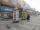Column with posters in the form of a cylinder is 2 m high and its diameter is 1.7 m. What is the content area for which it is possible to stick posters?
• Unknown number 24I think the number: a - is the same as the square area that has the 12th circumfence. What is this number? b - its half is 7 times bigger than its quarter. Is this the number?
• 3s prismIt is given a regular perpendicular triangular prism with a height 19.0 cm and a base edge length 7.1 cm. Calculate the volume of the prism.
• Collect rain waterThe garden water tank has a cylindrical shape with a diameter of 80 cm and a height of 12 dm. How many liters of water will fit into the tank?
• Spherical segmentThe spherical segment with height h=1 has a volume V=223. Calculate the radius of the sphere of which is cut this segment.
• Playground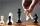On the special playground, there are 81 square sectors, each with a side of 5 m. How many players can fit on the playground if each player needs a 75 m2 area to play?
• Chord 3What is the radius of the circle where the chord is 2/3 of the radius from the center and has a length of 10 cm?
• Silo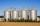Outer perimeter of silo is 32 m. Concrete wall is 35 cm thick. What is diameter and area of inside floor of silo?
• Concentric circlesIn the circle with diameter 19 cm is constructed chord 9 cm long. Calculate the radius of a concentric circle that touches this chord.
• TrapeziumThe area of trapezium is 35 cm2. Find its altitude if the bases are 6cm and 8 cm.
• Drawer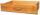The rectangular face of a drawer has a perimeter of 108 cm and a width of 18cm. Find the length and the area of the rectangular face of the drawer.
• The terrace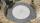Around the round pool with a diameter of 5.5 meters is a wooden terrace with a width of 130 cm. What is the area of the terrace?
• Hexa prismDetermine the volume of hex prism with edge base 4 cm. The body height is 28 cm.
• Angles in ratioThe size of the angles of the triangle are in ratio x: y = 7: 5 and the angle z is 42° lower than the angle y. Find size of the angles x, y, z.
• Sphere and coneWithin the sphere of radius G = 33 cm inscribe the cone with the largest volume. What is that volume, and what are the dimensions of the cone?
• Prism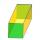The lenght, width and height of a right prism are 17, 11 and 11 respectively. What is the lenght of the longest segment whose endpoints are vertices of the prism?
• Rhombus ABCDRhombus ABCD, |AC| = 90 cm, |BD| = 49 cm. Calculate the perimeter of the rhombus ABCD.
• MediansCalculate the sides of a right triangle if the length of the medians to the legs are ta = 21 cm and tb=12 cm.
• 22/7 circleCalculate approximately area of a circle with radius 20 cm. When calculating π use 22/7.

Do you have an interesting mathematical word problem that you can't solve it? Submit a math problem, and we can try to solve it.

We will send a solution to your e-mail address. Solved examples are also published here. Please enter the e-mail correctly and check whether you don't have a full mailbox.

Please do not submit problems from current active competitions such as Mathematical Olympiad, correspondence seminars etc...